# 一文搞懂线性表(顺序表、链表)

### 前言

• 线性表：逻辑结构， 就是对外暴露数据之间的关系，不关心底层如何实现，数据结构的逻辑结构大分类就是线性结构和非线性结构而顺序表、链表都是一种线性表。

• 顺序表、链表：物理结构，他是实现一个结构实际物理地址上的结构。比如顺序表就是用数组实现。而链表用指针完成主要工作。不同的结构在不同的场景有不同的区别。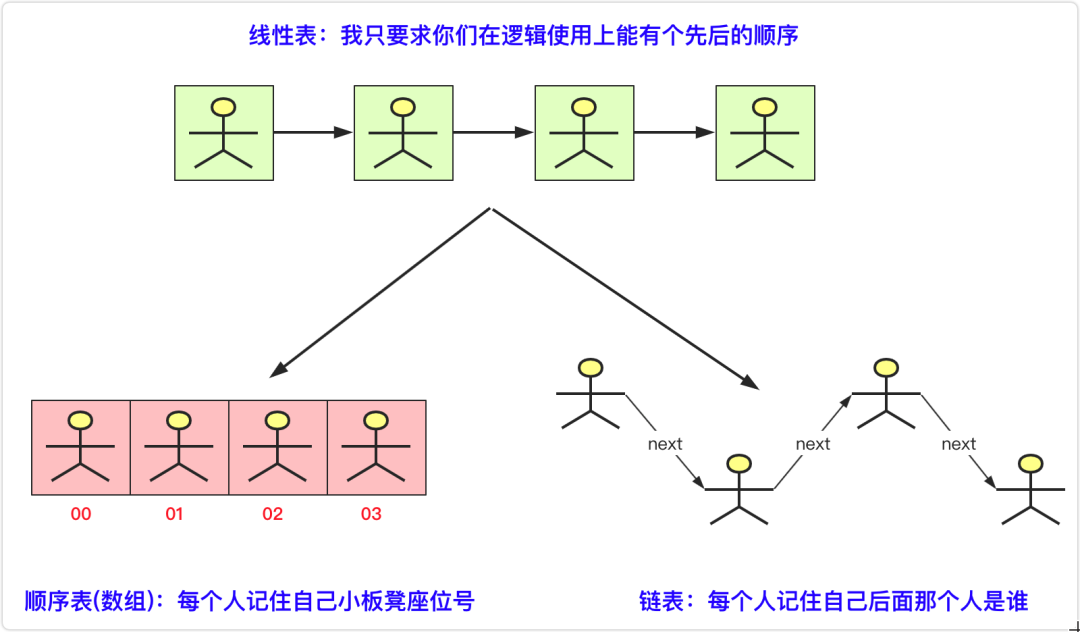### 线性表基本架构

``package LinerList;public interface ListInterface<T> {        void Init(int initsize);//初始化表    int length();    boolean isEmpty();//是否为空    int ElemIndex(T t);//找到编号    T getElem(int index) throws Exception;//根据index获取数据    void add(int index,T t) throws Exception;//根据index插入数据    void delete(int index) throws Exception;    void add(T t) throws Exception;//尾部插入    void set(int index,T t) throws Exception;    String toString();//转成String输出  }``

### 顺序表

#### 插入操作

add(int index,T t)

(1)从后(最后一个有数据位)向前到index依次后移一位，腾出index位置的空间

(2)将待插入数据赋值到index位置上，完成插入操作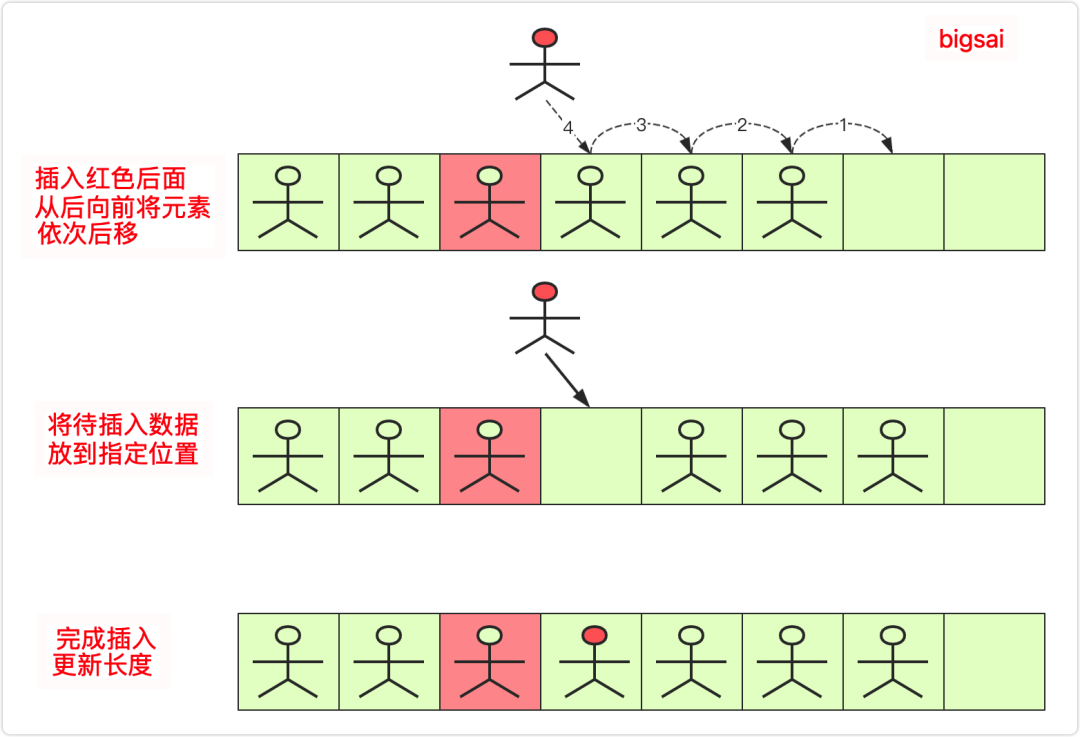#### 删除操作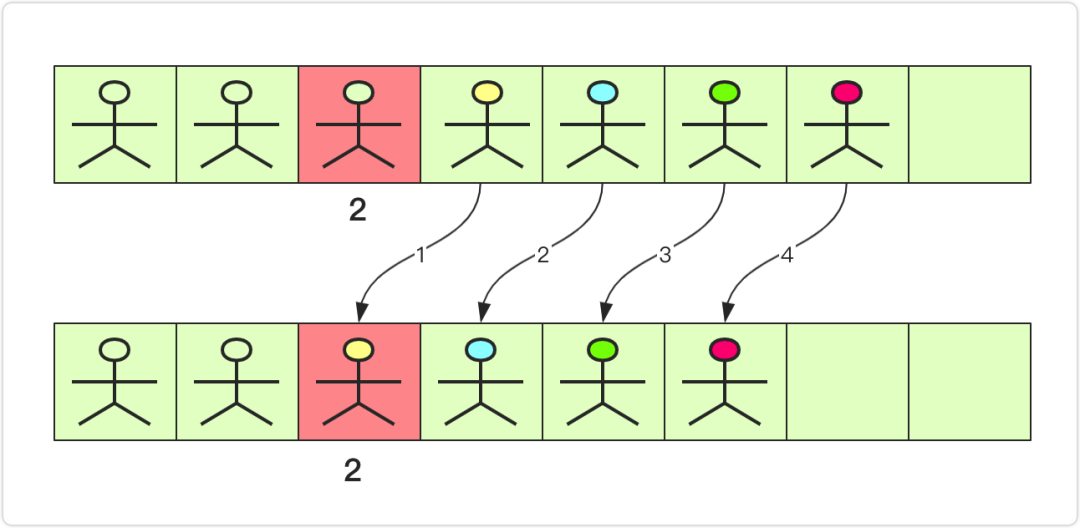#### 代码实现

``package LinerList;public class seqlist<T> implements ListInterface<T> {    private Object[] date;//数组存放数据    private int lenth;    public seqlist() {//初始大小默认为10        Init(10);    }    public void Init(int initsize) {//初始化        this.date=new Object[initsize];        lenth=0;            }    public int length() {               return this.lenth;    }    public boolean isEmpty() {//是否为空        if(this.lenth==0)            return true;        return false;    }    /*     * * @param t        * 返回相等结果，为-1为false     */    public int ElemIndex(T t) {        // TODO Auto-generated method stub        for(int i=0;i<date.length;i++)        {            if(date[i].equals(t))            {                return i;            }        }        return -1;    }    /*     *获得第几个元素     */    public T getElem(int index) throws Exception {        // TODO Auto-generated method stub        if(index<0||index>lenth-1)            throw new Exception("数值越界");        return (T) date[index];    }    public void add(T t) throws Exception {//尾部插入         add(lenth,t);    }    /*     *根据编号插入     */    public void add(int index, T t) throws Exception {        if(index<0||index>lenth)            throw new Exception("数值越界");        if (lenth==date.length)//扩容        {            Object newdate[]= new Object[lenth*2];            for(int i=0;i<lenth;i++)            {                newdate[i]=date[i];            }            date=newdate;        }        for(int i=lenth-1;i>=index;i--)//后面元素后移动        {            date[i+1]=date[i];        }        date[index]=t;//插入元素        lenth++;//顺序表长度+1    }    public void delete(int index) throws Exception {        if(index<0||index>lenth-1)            throw new Exception("数值越界");        for(int i=index;i<lenth;i++)//index之后元素前移动        {            date[i]=date[i+1];        }        lenth--;//长度-1      }    @Override    public void set(int index, T t) throws Exception {        if(index<0||index>lenth-1)            throw new Exception("数值越界");        date[index]=t;    }    public String  toString() {        String vaString="";        for(int i=0;i<lenth;i++)        {            vaString+=date[i].toString()+" ";        }        return vaString;    }}``

### 链表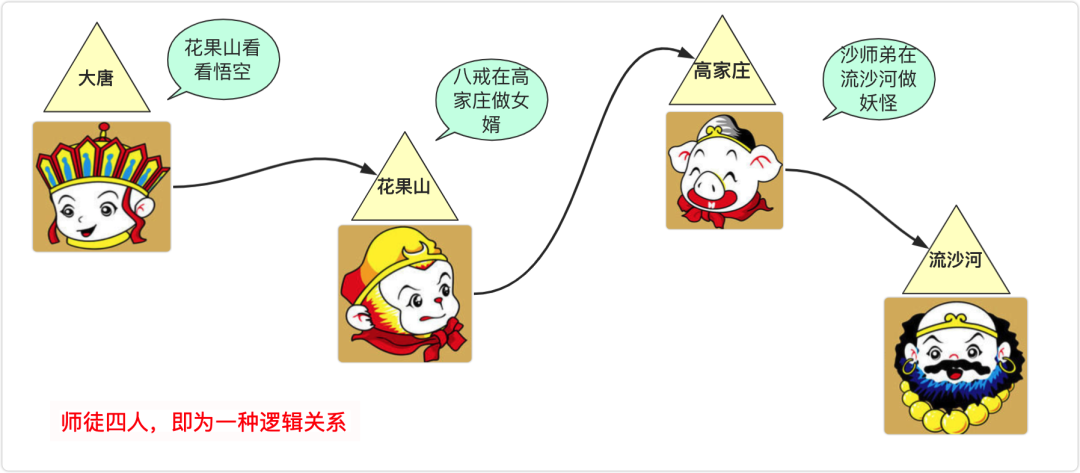#### 基本结构

``class node<T>{    T data;//结点的结果    node next;//下一个连接的结点    public node(){}    public node(T data)    {        this.data=data;    }    public node(T data, node next) {        this.data = data;        this.next = next;    } }``

#### 带头结点链表VS不带头结点链表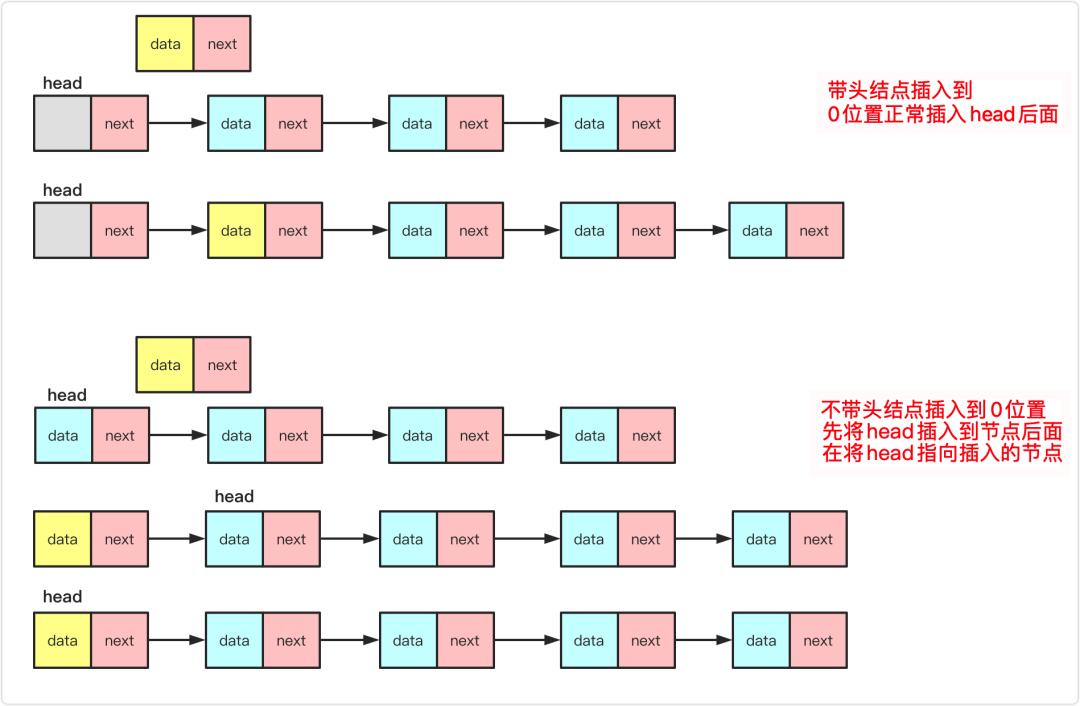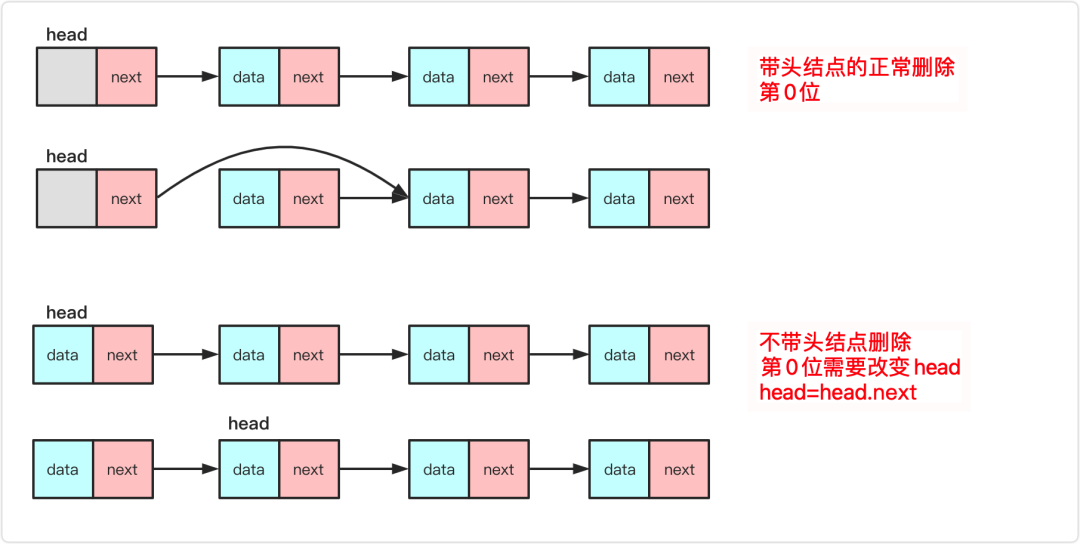#### 带头指针VS带尾指针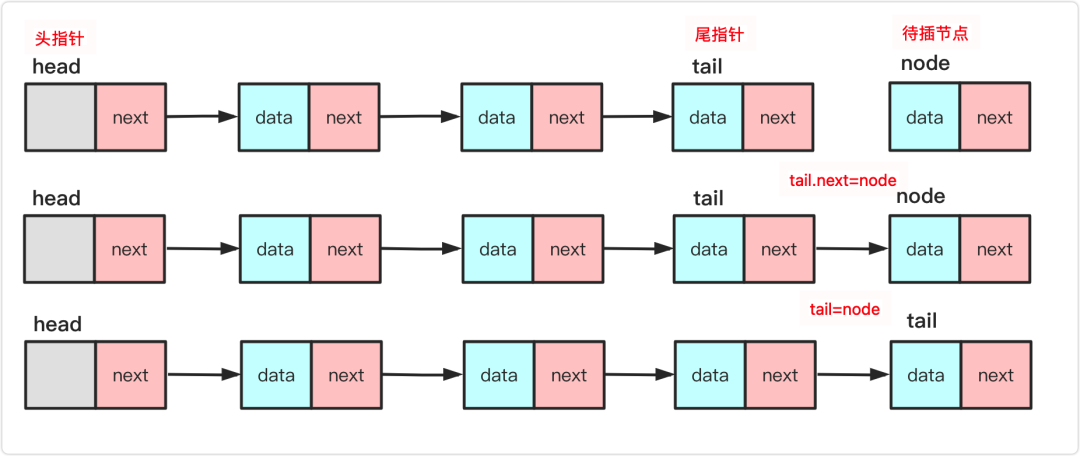#### 插入操作

add(int index,T t)

1. `node.next=pre.next`，将插入结点后面先与链表对应部分联系起来。此时node.next和pre.next一致。

2. `pre.next=node` 将node结点插入到链表中。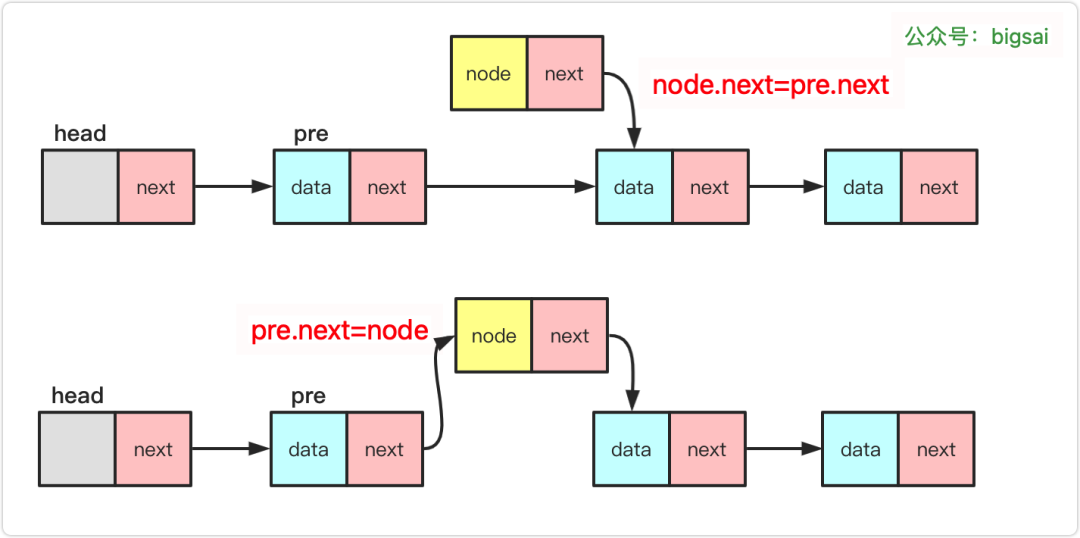#### 删除操作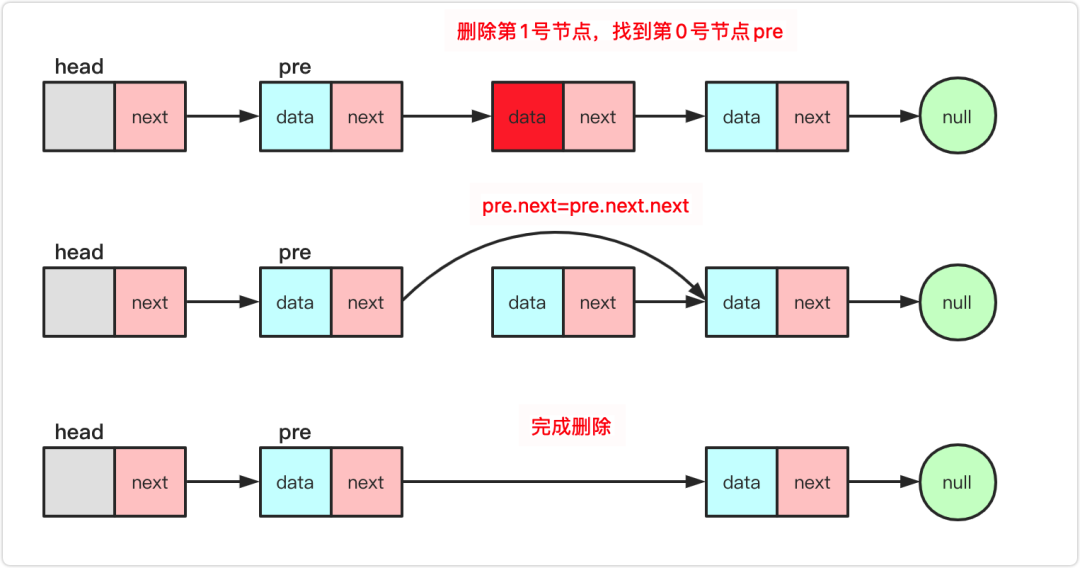#### 代码实现

``package LinerList;class node<T>{    T data;//结点的结果    node next;//下一个连接的结点    public node(){}    public node(T data)    {        this.data=data;    }    public node(T data, node next) {        this.data = data;        this.next = next;    }}public class Linkedlist<T> implements ListInterface<T>{    node head;    private int length;    public Linkedlist() {        head=new node();        length=0;    }    public void Init(int initsize) {        head.next=null;    }    public int length() {        return this.length;    }    public boolean isEmpty() {        if(length==0)return true;        else return false;    }    /*     * 获取元素编号     */    public int ElemIndex(T t) {        node team=head.next;        int index=0;        while(team.next!=null)        {            if(team.data.equals(t))            {                return index;            }            index++;            team=team.next;        }        return -1;//如果找不到    }    @Override    public T getElem(int index) throws Exception {        node team=head.next;        if(index<0||index>length-1)        {            throw new Exception("数值越界");        }        for(int i=0;i<index;i++)        {            team=team.next;        }        return (T) team.data;    }    public void add(T t) throws Exception {        add(length,t);    }    //带头结点的插入，第一个和最后一个一样操作    public void add(int index, T value) throws Exception {        if(index<0||index>length)        {            throw new Exception("数值越界");        }        node<T> team=head;//team 找到当前位置node        for(int i=0;i<index;i++)        {             team=team.next;        }        node<T>node =new node(value);//新建一个node        node.next=team.next;//指向index前位置的下一个指针        team.next=node;//自己变成index位置            length++;    }    @Override    public void delete(int index) throws Exception {        if(index<0||index>length-1)        {            throw new Exception("数值越界");        }        node<T> team=head;//team 找到当前位置node        for(int i=0;i<index;i++)//标记team 前一个结点        {             team=team.next;        }        //team.next结点就是我们要删除的结点        team.next=team.next.next;        length--;    }    @Override    public void set(int index, T t) throws Exception {        // TODO Auto-generated method stub        if(index<0||index>length-1)        {            throw new Exception("数值越界");        }        node<T> team=head;//team 找到当前位置node        for(int i=0;i<index;i++)        {             team=team.next;        }        team.data=t;//将数值赋值，其他不变    }    public String toString() {        String va="";        node team=head.next;        while(team!=null)        {            va+=team.data+" ";            team=team.next;        }        return va;    }}``

### 总结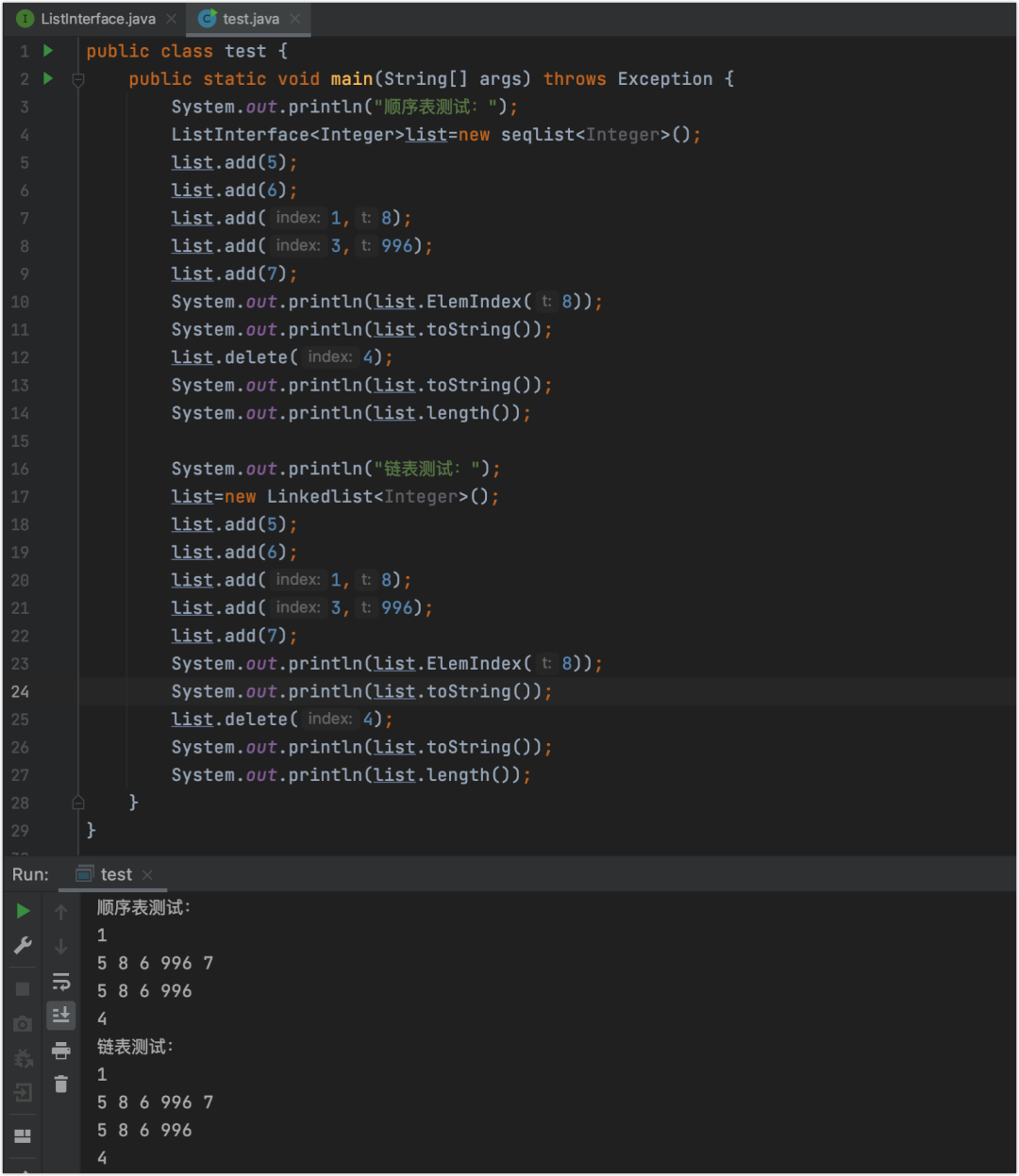Java中的Arraylist和LinkedList就是两种方式的代表，不过LinkedList使用双向链表优化，并且JDK也做了大量优化。所以大家不用造轮子，可以直接用，但是手写顺序表、单链表还是很有学习价值的。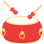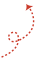「其他文章」